# Difference between revisions of "Pfaffian structure"

distribution

A vector subbundleof the tangent bundle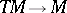of a manifold. The dimensionof the fibres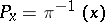is called the dimension of the Pfaffian structure, and the number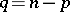(where) is called the rank or codimension. A Pfaffian structure of dimensioncan be considered as a field of-dimensional subspaces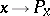on the manifold.

Usually a Pfaffian structure is given by a system of Pfaffian equations (cf. Pfaffian equation)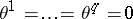or, dually, by indicating vector fields whose values at an arbitrary point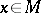form a basis of the subspace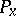.

A submanifold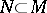is called an integral manifold of the Pfaffian structure if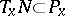for all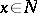. A Pfaffian structure is said to be completely integrable if through each pointthere passes a-dimensional integral manifold or, what is equivalent, if it can be locally given by a system of Pfaffian equations, where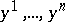are local coordinates in. This concept corresponds to the concept of a completely-integrable system of Pfaffian equations. Let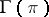be the space of sections of the bundle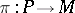(cf. also Section of a mapping) and letbe the space of differential-forms (cf. Differential form) which vanish on. According to Frobenius' theorem the Pfaffian structureis completely integrable if and only if the spaceis a subalgebra of the Lie algebra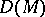of vector fields onor, what is equivalent, if the ideal generated by the space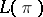in the algebraof differential forms is closed with respect to exterior differentiation.

Let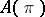be the Lie algebra of infinitesimal automorphisms of the Pfaffian structure, that is, the set of vector fields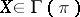for which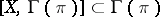. The algebrais a subalgebra of the Lie algebraand at the same time a module over the ring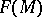of smooth functions on. The quotient modulecharacterizes the degree of non-integrability of the Pfaffian structure.

The Pfaffian structureis regular if the dimension of the space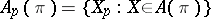does not depend on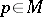. In this caseis the space of sections of a completely-integrable Pfaffian structure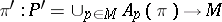, called the characteristic system of the Pfaffian structure. The rank of the structure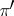is called the class of the Pfaffian structure, and it is equal to the smallest possible number of coordinates of a local coordinate system in which all-forms incan be expressed. The class of a regular Pfaffian structure of rank 1 (that is, a field of hyperplanes) is odd and forms a complete system of local invariants: In a local coordinate system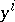a Pfaffian structure of class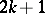is locally given by a Pfaffian equation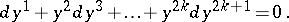Another important local invariant of the Pfaffian structure is its genus, which indicates the dimension of the maximal integral non-singular manifolds (see Pfaffian problem). A complete system of local invariants of a Pfaffian structure of dimensionwhen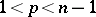is unknown.

A Pfaffian structure can be considered as a-structure of infinite type, whereis the group of linear transformations of the spaceleaving invariant a-dimensional coordinate plane. Its first-order structure function corresponds to an-bilinear mapping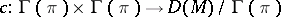, defined by the commutation of vector fields. The spacecoincides with the kernel of the vector-valued bilinear form.

For references see Pfaffian problem.

How to Cite This Entry:
Pfaffian structure. Encyclopedia of Mathematics. URL: http://encyclopediaofmath.org/index.php?title=Pfaffian_structure&oldid=13963
This article was adapted from an original article by D.V. Alekseevskii (originator), which appeared in Encyclopedia of Mathematics - ISBN 1402006098. See original article